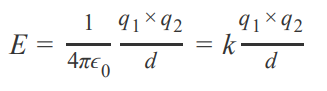# Problem: The formation of CsCl from Cs(s) and Cl2 (g) involves the following steps:1. Cs(s) → Cs(g)2. 1/2 Cl2 (g) → Cl(g)3. Cs(g) → Cs+(g) + e−4. Cl(g) + e− → Cl−(g)5. Cs+(g) + Cl−(g) → CsCl(s)Which of these steps absorb energy and which release energy?The electrostatic attraction between oppositely charged ions is called an ionic bond. The net energy change in the formation of an ionic bond is a composite of five steps, some of which absorb energy and some of which release energy. The steps are summarized for a generic metal, M, and a diatomic halogen, X2 , as1. sublimation of the metal2. dissociation of the X−X bond,3. ionization of the metal,4. transfer of the electron to X, and5. crystalline solid formation.The overall energy transfer is the sum of the energy changes for each step. The magnitude of the potential energy of two interacting charges, EE, (which is related to Lattice energy) is the energy associated with the strength of the attraction between ions in the ionic compound and can be determined bywhere k is a constant equal to 1/(4πϵ0) = 1/(1.11 × 10 − 10 C2 /(J ⋅ m)), q1 and q2 are the charges of the ions, and dd is the distance between the nuclei of the ions.

###### FREE Expert SolutionView Complete Written Solution
###### Problem Details

The formation of CsCl from Cs(s) and Cl2 (g) involves the following steps:

1. Cs(s) → Cs(g)

2. 1/2 Cl2 (g) → Cl(g)

3. Cs(g) → Cs+(g) + e

4. Cl(g) + e → Cl(g)

5. Cs+(g) + Cl(g) → CsCl(s)

Which of these steps absorb energy and which release energy?

The electrostatic attraction between oppositely charged ions is called an ionic bond. The net energy change in the formation of an ionic bond is a composite of five steps, some of which absorb energy and some of which release energy. The steps are summarized for a generic metal, M, and a diatomic halogen, X2 , as

1. sublimation of the metal
2. dissociation of the X−X bond,
3. ionization of the metal,
4. transfer of the electron to X, and
5. crystalline solid formation.

The overall energy transfer is the sum of the energy changes for each step. The magnitude of the potential energy of two interacting charges, EE, (which is related to Lattice energy) is the energy associated with the strength of the attraction between ions in the ionic compound and can be determined bywhere k is a constant equal to 1/(4πϵ0) = 1/(1.11 × 10 − 10 C2 /(J ⋅ m)), q1 and q2 are the charges of the ions, and dd is the distance between the nuclei of the ions.